Publish Date: Nov 26, 2018 | 79 Ratings | 4.46 out of 5 | Print | 9 Customer Reviews | Submit your review

## Overview

This article discusses two common zero padding applications, including speeding up fast Fourier transform (FFT) calculation and the perceived benefit of improved resolution in the results. To complement the text, the article includes several LabVIEW 6.1 examples and tools to explain some of the ideas.

### 1. Introduction

If you have ever worked with the nuts and bolts of computer-based spectral analysis, you have likely encountered zero padding. Often applied as a step in the signal processing associated with spectral analysis, zero padding involves appending one or more zeros to the end of a signal. It is a tool with a high perceived value related to improving the speed and resolution of the results. Unfortunately, it can lead to results that are easy to misinterpret.

This article discusses two common zero padding applications, including speeding up fast Fourier transform (FFT) calculation and the perceived benefit of improved resolution in the results. To complement the text, the article includes live LabVIEW 6.1 examples and tools to explain some of the ideas. The section titled "Zero Padding with LabVIEW" discusses the mechanics of zero padding using LabVIEW and describes these examples. Follow the links in the section to download the software and run the examples as you read the text.

Before getting to the details, consider where zero padding fits as one of the steps in a frequency-domain analysis algorithm. In a real application, the high-level steps described in this figure would likely require some additional processing to get a usable result. Examples of such extra processing might include vertical scaling associated with applying a window or buffering associated with performing a running FFT on a live signal. It is important to note that if you want to apply a window to your input signal, you should do so before appending any zeros.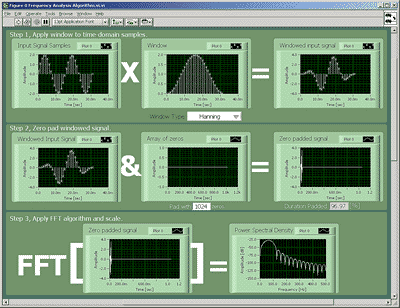Zero padding is often a step in the signal processing associated with spectral analysis.

### 2. Zero Padding Now Optional

Many engineers have experience with zero padding because of the nature of some FFT algorithms used to transform an array of data into spectral information. Some efficient FFT algorithms require an input array that is a power-of-two length. When applying these algorithms to non-power-of-two length datasets, you must zero pad or truncate your dataset to a power-of-two length.

Because some modern FFT algorithms do not require a power-of-two length data set, the power-of-two length rule is no longer a requirement. However, power-of-two algorithms are typically more efficient in terms of the number of FFTs that you can calculate per second. This difference in efficiency can be the deciding factor for some applications -- the choice could depend on which of these options requires less processing:

• Data manipulation related to zero-padding + efficient FFT algorithm on a power-of-two length dataset
• Less efficient FFT algorithm on a nonpower-of-two length dataset

The ever-increasing speed of modern processors simplifies the choice somewhat because the rate of calculating an FFT can increase without changing algorithms or block lengths. Nonetheless, few would argue that efficiency would ever be a nonissue because it enables other improvements in your measurement applications. If you can implement a more efficient FFT in your measurement code, you will have more processor time to dedicate to new features.

### 3. Padded Records Not Always a Good Fit

Differences in efficiency are not the only reason to change the input dataset length. Another issue involves how the content of the signals you are examining interacts with the discrete, or sampled, nature of the measurement and the computer-based operations that you apply to it. For the case where you know frequency interest for your input signal, you can choose an appropriate combination of sampling rate and record length to ensure that the FFT output will include that frequency.

Consider that the output of the FFT is an array of values that represent what is actually a continuous (analog) spectrum. More accurately, an FFT is an efficient implementation of a discrete Fourier transform (DFT), an operation that results in samples of a discrete time Fourier transform (DTFT). The values in this array represent samples that are evenly distributed over a predefined frequency range. Each value represents a level at a specific frequency and this frequency is set by the following two constants:

• The sampling rate of the input signal (fs)
• The number of input samples (N)

Given N samples of an input signal sampled at a rate of fs, the FFT output will be an array with N/2+1 samples. The elements of this array, which are also known as bins, will distribute evenly in frequency between 0 and fs / 2. Because the input array represents a duration of time equal to N / fs, the equal spacing between the output bins must be 1 / N / fs = fs / N. With this spacing, slot n of the FFT output array represents the frequency n (fs/N). The idea is to choose values for fs and N such that some value of n (fs / N) will equal your frequency of interest.

### 4. Bin Shift Problems

Zero padding shifts the inter-sample spacing in frequency of the array that represents your result. This shift can cause problems if it alters the array positions relative to the frequency of interest. The zero padding figure shows an example where the frequency of interest in the acquired signal is known to be 100 [Hz]. In the top window of the figure, applying an FFT to 100 samples of a signal acquired at a sampling rate of 10k [samples / s] results in an array of 50 values with the bin at array slot 10 conveniently representing the signal level at 100 [Hz].

To see the problem, consider what happens if you decide to zero pad the input signal with 28 to boost the length to a power of two. The fact that you no longer have a bin at exactly 100 [Hz] outweighs the benefit of any efficiency gain. As shown on the lower window the zero padding figure, the frequency-domain information related to the 100-[Hz] signal component is now spread between the 64 samples that result from the 128-point FFT. Information about the original 100 [Hz] signal component remains buried in the output samples. The computational overhead associated with any processing you apply to extract it will likely outweigh any benefits you might have garnered from having a power-of-two input length.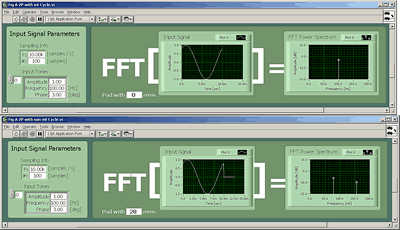When you apply an FFT to a record length that covers an integer number of cycles at the frequency of interest, the bins of the resulting FFT will align with that frequency.

### 5. Does Signal = Signal + 0?

To better understand the effect on the frequency-domain sample spacing when you append zeros to the input, you can plug the changes into the above-mentioned formulas. When you append Nz zeros to an input signal with N samples, the FFT output becomes (N + Nz)/2 +1 samples. Because appending zeros does not change the input sampling rate, the frequency span of the FFT output will remain the same. The new output remains an evenly distributed set of samples spread over 0 to fs / 2. The sample spacing of the new output must decrease to fit more samples over the same frequency range. The slots of the output array now represent frequencies equal to n (fs / (N + Nz)), where n is again the index of the array.

The decreased spacing is in the sampled output of the FFT and corresponds to a resolution increase in those samples. It is important to note that this increase does not improve frequency resolution of your analysis of the input signal.

### 6. Distinguishing Resolutions

In this situation, you can distinguish two separate frequency resolutions. The first is the resolution that quantifies the ability to distinguish two closely spaced frequencies from your original input signal. The second is the inter-sample spacing of the array of values that is output from an FFT-based algorithm.

The resolution that distinguishes closely spaced frequencies is often the one that is of interest in your analysis because it is relevant to the spacing of frequencies from the original input signal. It is common for this to become confusing because these resolutions are equal if you have not applied any zero padding.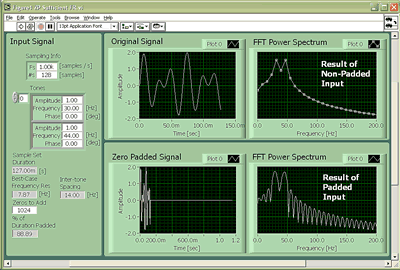Sufficient frequency resolution allows you to distinguish closely spaced peaks with or without zero padding.

The sufficient frequency resolution image provides an example of applying an FFT power spectrum to padded and nonpadded versions of an input signal that contains two main frequency components. The sampling rate and duration of the input signal are such that the frequency-distinguishing resolution is sufficient to distinguish the two components. The FFT Power Spectrum results from the padded and nonpadded inputs show clear peaks at each frequency.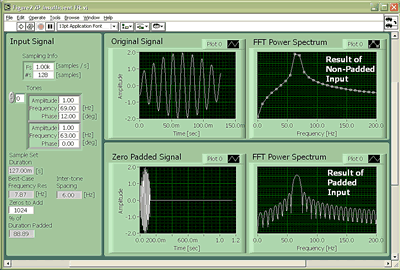Padding does not help distinguish closely spaced peaks when your original input signal lacks sufficient frequency domain resolution.

The figure above presents the case where the input signal lacks sufficient frequency distinguishing resolution. As the figure shows, the padded and nonpadded results show no hint at two peaks.

Zero padding does not improve the resolution associated with your input signal -- the only way to do this is to increase the time duration of the block of signal that you examine. It is useful to remember that the best-case frequency resolution associated with the content of the signal that you measure is 1/T, where T is the time duration that your original, or nonpadded input samples cover.

### 7. Zero Padding with LabVIEW

As a general-purpose programming language, LabVIEW offers unlimited possibilities for zero padding associated with spectral analysis. This section narrows the possibilities down to a select subset. Download the examples discussed in this section.

Perhaps the most obvious is the built-in subVI from the array-based signal processing palette, "Zero Padder":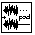This VI is a good choice for efficiently increasing an array length to the next higher power-of-two. The benefit is that the array-based FFT algorithms that ship with LabVIEW Full or Pro are more efficient with array lengths that are equal to a power of two.

Padding an array with zeros to an arbitrary length requires a custom solution. The VI "zeroPadArrayarbitraryLength.vi" from the library associated with this article does the job for an array, and the code is straightforward. If you are new to LabVIEW, note the use of the initialize array operation in the LabVIEW code for this VI. It is an efficient alternative to building an array on the border of a For loop.

If you are working with LabVIEW 6i (or higher) and familiar with the waveform data type (WFD) that was new to that release, you will note that the "Zero Padder" is designed for array-based inputs and outputs. The VIs in the attached library, "subPadWFDarbitrarylength.vi" and "subPadWFDPowerofTwoLength.vi" do the job for the WFD. The LabVIEW code is straighforward: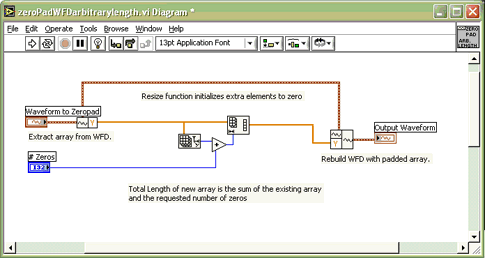When you work with the WFD, you want to utilize the high-level tools for spectral analysis including the FFT Power Spectrum and several others. These VIs will not realize their inputs have been zero padded, so you will need to tweak them in certain circumstances.

One area that you need to consider involves windowing. Enabling the built-in windowing capabilities for any of these VIs applies the window to the entire block of the input signal. As such, you will not want to apply it to a block that you have padded. To deal with this solution, you can ignore the windowing capabilities of these tools and apply any required windowing externally, as shown in the example VI, "WFDPSDExample.vi."

Because zero padding does not affect the input sampling rate, these VIs automatically handle frequency scaling after padding. If you examine "WFDPSDExample.vi", you will see that the LabVIEW high-level FFT Power Spectral Density vi automatically handles the x-axis scaling. (The other high-level FFT-based WFD spectral analysis VIs can also handle this scaling.)

I would like to thank several other engineers here at National Instruments for their ideas and technical review of this article: Mike Cerna, Jim Lewis, Jim Nagle, and Yong Rao.

Customer Reviews
9 Reviews | Submit your review

Zero-padding causes ringing  - Feb 29, 2012

Zero padding a signal is the same as multiplying a box function with your signal, which translates to a convolution of a sinc with your signal in the frequency domain, which might cause ringing.

Zero padding is for amplitude accuracy, not frequency resolution  - Jun 15, 2011

Zero padding is used in spectral analysis with FFT's to improve the accuracy of the reported amplitudes, not to increase frequency resolution. Without zero- padding, input frequencies that lie between the FFt bins will be attenuated in the output. Zero padding in the time domain is equivalent to optimal interpolation in the frequency domain, which restores the correct amplitudes. See https://ccrma.stanford.edu/~jos/st/Spectru m_Analysis_Sinusoid_Windowing.html

Interpolation is in wrong   - Nov 19, 2010

Christian, what you're imagining is not without merit - but you're thinking in the wrong space. By interpolating values in between he known values you are increasing the sampling rate (you're sampling more often) and not increase the sampling range (how long you sampled). The result is that your computed spectrum will have higher frequency components, not more closely spaced frequency components. As a rule, to do this correctly means that you must have some information regarding these higher spectral components, which in general you do not - or you wouldn't need to do the experiment.

Energy wraparound  - Sep 21, 2010

One of the main reasons for zero padding is prevent energy wrap-around when filtering in the Fourier domain. The DFT assumes the sequence is cyclical, which means that strong energy at the end of the sequence can appear at the front of the sequence due to filtering, and vice-versa. In practice the sequences we are filtering are seldom cyclical. Zero padding is a way to prevent this, and thus reduce the side effects of the cyclicality assumption.

Linearly interpolating values  - Aug 11, 2010

First off, thanks a bunch for a very informative article! I am particularly interested in ways to increase the frequency resolution of the input signal without having to obtain a longer-time window of data. I understand the point about zero-padding being unable to achieve this for me, but what if, instead of appending zeroes to the end of the signal, I linearly interpolate new values in between existing values? I.e. I have a signal of {1,2,3,4}, and then I insert values to create the signal {1, 1.5, 2, 2.5, 3, 3.5, 4, 0}, ending with a single zero-pad to keep power of 2. This directly constitutes fabricating data, but if you know enough about how the signal in question generally behaves to be able to assume that actual values wouldn't have been far off if sampled, then can this help you improve the frequency resolution of the input signal without having to wait longer for the buffer to fill with values?

- Apr 1, 2010

Information is very useful! Thanks! Btw, it seems to me, that in section "Bin Shift Problems" the sampling frequency should be 1k [samples/second] in order to get 100Hz frequency responce on the 10th slot.

- Sep 9, 2009

Great info! Until now I thought zero padding improves resolution...:(. Thanks again!

good tutorial  - May 16, 2008

Good article. I've been having difficulty re-learning how to define frequency resolution. This is one of the few good references I found on the web.

Very Interesting, Good Info!  - Feb 12, 2003